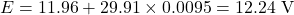## On a cold day a battery has a terminal voltage of Vi- 11.96 V and an internal resistance of ri = 0.0095 ? On a warm day the battery has a te

Question

On a cold day a battery has a terminal voltage of Vi- 11.96 V and an internal resistance of ri = 0.0095 ? On a warm day the battery has a terminal voltage of V2-1161 V and an internal resistance of r2 -0.021 2. The device it powers draws the same current at both tempertures. Assume the emf of the battery does not depend on temperature. 33% Part (a) Enter an expression for the current, I, in terms of the given quantities.Part (b) Calculate the cument, I, in amperes. 33% Part (c) what is the battery’s emf, in volts?

in progress 0
6 months 2021-09-05T00:20:34+00:00 1 Answers 2 views 0

(a)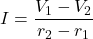(b) 29.91 A

(c) 12.24 V

Explanation:

(a) The emf of a battery is the sum of its terminal voltage and lost voltage. The lost voltage is due to the internal resistance. By this, we have for the first condition,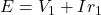E is the emf,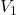is the terminal voltage and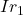is the lost voltage with I being the current.

Since the same current is drawn in both conditions and the emf does not depend on temperature, we have similar for the second condition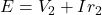Equating both equations and solving for I, we have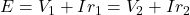(b) Substitute the values from the question into the expression for I in (a)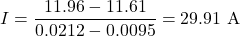(c) Substitute the value of I into any of the equations of E